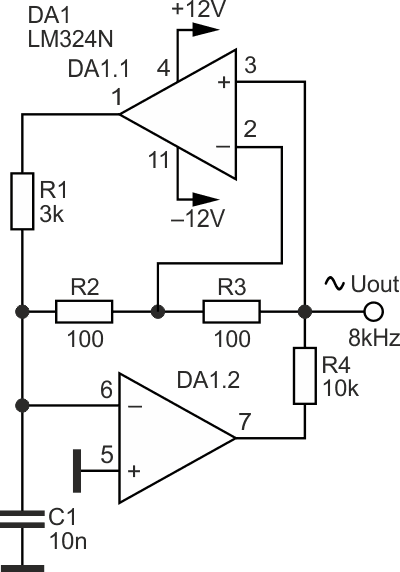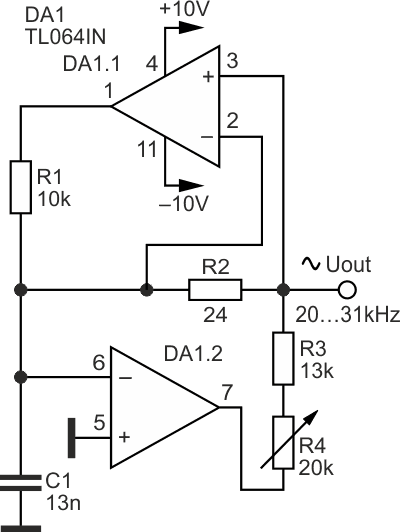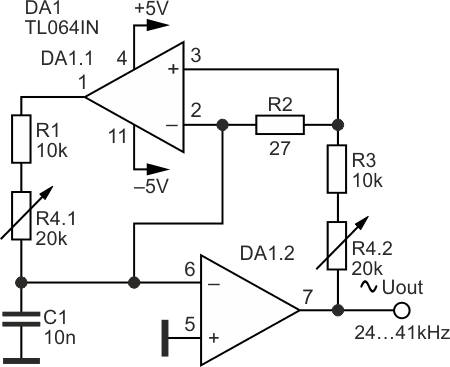# Single capacitor sine-wave RC-oscillators

## Texas Instruments LM324 TL064

Sine-wave RC-oscillators with smooth frequency tuning described in the article radically and favorably differ from previously known generators of this purpose by the presence of only one frequency-setting capacitor.Sine-wave RC-oscillators have been and remain one of the most popular devices in radio electronics. Despite its many decades of history, the circuitry technique of such generators has not undergone significant changes. In particular, any such generator requires at least two frequency-setting capacitors for its construction.

Figures 1-3 show schematics of simple sine-wave RC-oscillators made on a pair of operational amplifiers and containing, in addition to several resistors, only one frequency-setting capacitor.Figure 1. Sine-wave oscillator on the LM324N chip.

The RC oscillator in Figure 1 contains two operational amplifiers DA1.1 and DA1.2 of the LM324N IC. The oscillator operates at a fixed frequency of 8 kHz. The sinusoidal output voltage amplitude at a supply voltage of 12 V is 4.8 V with harmonic distortion of 1.26%.Figure 2. Sine-wave oscillator on the TL064IN chip.

The oscillator in Figure 2 uses the TL064IN IC. This circuit also differs from the previous one in the values of RC parts and supply voltage. With the help of the potentiometer R4, the generator is tuned in the frequency range from 20 to 31 kHz. In this case the harmonic distortion changes from 0.85 to 0.45%, and the output signals amplitude varies from 180 to 230 mV. Sinusoidal signals can also be taken directly from the outputs of operational amplifiers, for example, from the DA1.2 output, where the signal amplitude is 8.4 V but with harmonic distortion 1.32%.Figure 3. A variant of the sine-wave oscillator on the TL064IN chip.

The oscillator in Figure 3 uses a dual potentiometer R4.1, R4.2 to adjust the oscillation frequency. This oscillator operates in the frequency range of 24...41 kHz. The amplitude of the output signal depends little on the frequency. At a supply voltage of 5 V and a frequency of 24 kHz, the signal amplitude is 3.25 V (harmonic distortion is 0.05%); at a frequency of 41 kHz, the signal amplitude is 3.33 V (harmonic distortion is 0.64%).

The oscillator frequency in Figure 3 is inversely proportional to the square root of the C1(R1+R4.1) product. The value of the C1 capacitor should be in the range from 10 to 100 nF.

The disadvantage of oscillators discussed above is that the oscillation frequency depends markedly on the supply voltage. On the other hand, this property can be used for good – when creating oscillators with automatic frequency control or variable frequency oscillators controlled by changing the supply voltage.

## Materials on the topic

You may have to register before you can post comments and get full access to forum.
 User Name Remember Me? Password